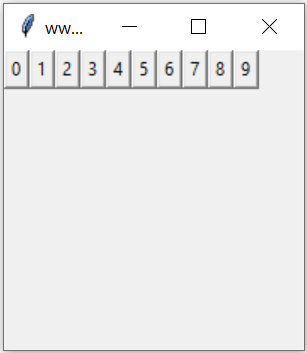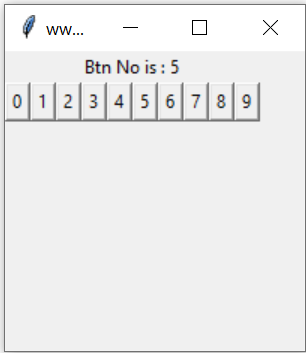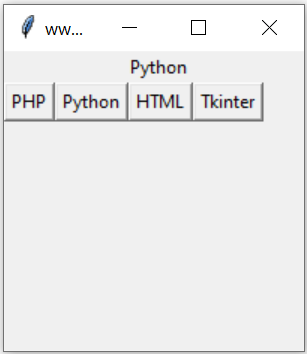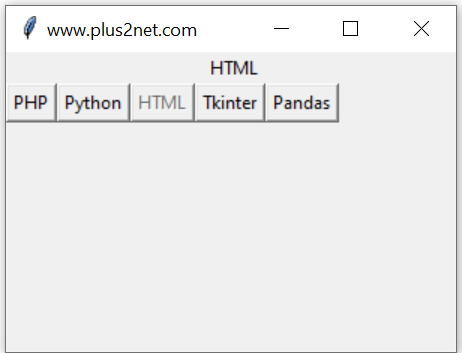# Dynamic Handling of buttons in Tkinter

Basics of Python Tkinter Button

Buttons can be created dynamically and can be referred for handling click events. Such requirements may occur when we are not sure how many buttons are required and how the events are to be handled.

## Creating buttons dynamicallyWe can set a variable to any integer and based on this value , number of buttons will be created. The text on the buttons should display the (unique ) number of the button.
``````import tkinter  as tk
from tkinter import *
my_w = tk.Tk()
my_w.geometry("200x200")  # Size of the window

n=10 # number of buttons
i=3
for j in range(n):
e = Button(my_w, text=j)
e.grid(row=i, column=j)

my_w.mainloop()  # Keep the window open``````

## Reading the data written on the buttonWe can read the value written on the button and display the same on button click. Note that the value we can’t use directly as it will always store the last value of the loop. So each button ( time ) we will assign the value to another variable to pass it through loop.
``````import tkinter  as tk
from tkinter import *
my_w = tk.Tk()
my_w.geometry("200x200")  # Size of the window

my_str = tk.StringVar()
l1 = tk.Label(my_w,  textvariable=my_str, width=10 )
l1.grid(row=1,column=2,columnspan=6)

def my_fun(k):
my_str.set("Btn No is : "+ str(k) )

n=10 # number of buttons
i=2
for j in range(n):
e = Button(my_w, text=j,command=lambda k=j: my_fun(k))
e.grid(row=i, column=j)

my_w.mainloop()  # Keep the window open``````

## Using string variablesWe will create one button for each element of a tuple of strings, on click of the button the string assigned to the button will be passed to another function and this function will display the string using one Label.
``````import tkinter as tk
my_w = tk.Tk()
my_w.geometry("200x200")  # Size of the window

my_str = tk.StringVar()
l1 = tk.Label(my_w,  textvariable=my_str, width=10 )
l1.grid(row=0,column=1,columnspan=5)

def show_lan(my_language):
my_str.set(my_language)

list_languages = ("PHP","Python","HTML","Tkinter")
var = 0

for language in list_languages:
btn = tk.Button(my_w, text=language, command=lambda lan=language:show_lan(lan))
btn.grid(row=1,column=var)
var += 1

root.mainloop()``````

## Disable Enable buttonsIn above code we will add a feature that after the click of the button the sting of the button will be displayed and the button will be disabled for further click. When some other button is clicked then the previous button will be enabled and the new button will be disabled.

To do this we have to store the reference of the buttons in a list as these buttons are created dynamically. This list will be used to loop through and enable or disable each buttons by using the value of var ( the button which is clicked )
``````import tkinter as tk
my_w = tk.Tk()
my_w.geometry("300x200")  # Size of the window

my_str = tk.StringVar()
l1 = tk.Label(my_w,  textvariable=my_str, width=10 )
l1.grid(row=0,column=2,columnspan=6)

def show_lan(my_language,var):
my_str.set(my_language)
#loop through all the buttons to enable or disable each one
for i in range(len(buttons)):
if i==var:
buttons[i].config(state="disabled")
else:
buttons[i].config(state="normal")

list_languages = ("PHP","Python","HTML","Tkinter","Pandas")
var = 0

buttons = [] # to store button references
#command=lambda index=index, n=n: appear(index, n)
for language in list_languages:
btn = tk.Button(my_w, text=language, command=lambda var=var,lan=language:show_lan(lan,var))
btn.grid(row=1,column=var)
var += 1
root.mainloop()``````plus2net.com